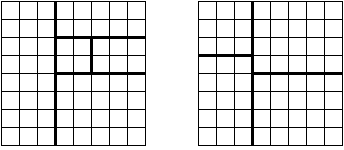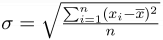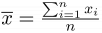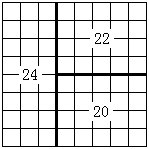## Day 2, Problem 1 - Chessboard Division

An 8×8 chessboard is divided as follows: Cut out one rectangular section such that the remaining section is also rectangular. Continue cutting the remaining section again in this fashion. After making (n − 1) cuts, there should be n remaining rectangular sections of the chessboard. Each cut may only be made on grid-lines of the board.The first figure above is an example of an acceptable cutting method, while the second figure above is an example of an unacceptable cutting method.

Each cell in the original chessboard has a score value, and the score value of any rectangular division is the sum of the scores of all of the cells that it contains. Now we must divide the 8×8 chessboard into n rectangular sections using the method above, while minimizing the mean squared error of the scores of each section.

The mean squared error (MSE)$\textstyle \sigma = \sqrt{\frac{\sum_{i=1}^{n}(x_i-\overline{x})^2}{n}}$, where the mean$\textstyle \overline{x}=\frac{\sum_{i=1}^{n}x_i}{n}$, and xi represents the score of the i-th board division.

Write a program that, given the scores of cells on the chess board and the number of divisions n, finds the minimum possible value of σ.

### Input Format

The first line of input contains the integer n (1 < n < 15). Line 2 to line 9 of input each contain 8 non-negative space-separated integers less than 100, describing the score values of each cell on the chessboard.

### Output Format

The output should contain the single number σ, rounded and displayed to three digits after the decimal point.

### Sample Input

3
1 1 1 1 1 1 1 3
1 1 1 1 1 1 1 1
1 1 1 1 1 1 1 1
1 1 1 1 1 1 1 1
1 1 1 1 1 1 1 1
1 1 1 1 1 1 1 1
1 1 1 1 1 1 1 0
1 1 1 1 1 1 0 3


### Sample Output

1.633Point Value: 15 (partial)
Time Limit: 1.00s
Memory Limit: 16M